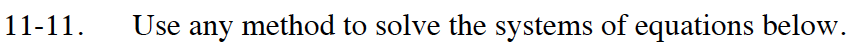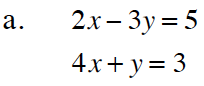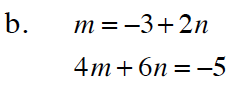Home > CC4 > Chapter 11 > Lesson 11.1.1 > Problem11-11

11-11.
1. Use any method to solve the systems of equations below. Homework Help ✎

2.  a. 2x – 3y = 5 4x + y = 3 b. m = –3 + 2n 4m + 6n = –5(−2, 0.5)